# DMelt:Plots/2 Plots in 2D

Limitted access. First login to DataMelt if you are a full DataMelt member. Then login to HandWiki as a user.

# Plots using MatLab syntaxjhplot.Plot is a simplest canvas to show data and functions. It is less Java oriented, but more oriented towards MatLab syntax (which does not assume operation with objects and dynamical access of methods).

from jhplot  import *

j=Plot()
j.plot([-1,2,3], [0.5, 0.2, 0.3])
j.show()
j.export("a.pdf")   # save as PDF file
# j.export("a.eps") # save as EPS (PostScript) format
# j.export("a.svg") # save as SVG file

Here we created X-Y plot and saved as PDF image. You can also use the "savefig()" method, which is equivalent to the export method.

# Interactive 2D canvases

Below we discuss more complicated plot canvases which can be used to display data in 2D. Such canvases usually have the names starting with the capital "H". Typically, you can build a plot to show 2D data as this:

from jhplot *
c1 = HPlot("Canvas",600,400,2,1) # canvas size 600x400, 2 plot regions
c1.visible(100,200)  # make it visible and position at 100 , 200 pixels on the screen
c1.setAutoRange()    # autorange for X
c1.draw(object1)     # draw object1 (H1D,F1D,P1D etc)
c1.draw(object2)     # draw a new object
c1.export("figure.pdf") # export to PDF file

This code create a canvas with the size 600x400 (in pixels), it has 2 pads to show data. The method visible(100,200) make the canvas visible and sets its location on the screen at position 100 (in X from left conner) and 200 (from top) in pixels. If you want a default position, jut call "visible()". Then you can draw any mathematical object or data. Then you can export the image to vector format. Now you are ready to plot functions, histograms and datasets. See thejhplot.HPlot documentation. It should be noted thejhplot.HPlot canvas can be replaced by any other canvas described above.

## Several plotting regions

These canvases can be used to show several pads (plot regions) with plotted objects. Specify the number of divisions in X and Y in the constructors. The navigate to a specific plot region using the method "cd()". Here is example of how to create 2x2 pads on a single canvas:

from jhplot  import *

c1 = HPlot("Canvas",500,400,2,2)
c1.visible()
c1. setRangeAll(0,10,0,10)
h1 = P1D("Simple")

c1.cd(1,1)

c1.cd(1,2)

c1.cd(2,1)

c1.cd(2,2)
c1.export ("example.pdf") # export to PDF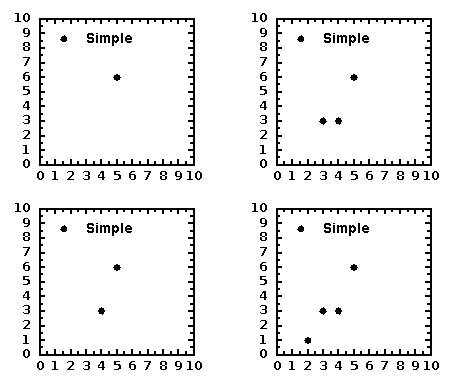Here we use the same X and Y ranges. One can use "setAutoRange()" (after each "cd()") method to set autorange for each pad. Also, try "setRange(xmin,xmax,ymin,ymax)" to set fixed ranges for each pads. it shows 4 pads with data points.

The plots are fully customizable, i.e. one can change any attribute (axis, label, ticks, ticks label).

## Exporting to images

When DMelt functions, histograms, data structures are shown, one can create an image using the menu [File]-[Export]. One can also save image in a file using the method "export(file)" to a variety of vector graphics formats as well as bitmap image formats.

In the example below, we save an instance of the HPlot class to a PDF file:

c1.export('image.pdf')

we export drawings on the canvas HPlot (c1 represents its instance) into a PDF file. One can also export it into PNG, JPEG, EPS, PS etc. formats using the appropriate extension for the output file name.

As example, simply append the statement "c1.export('image.ps')" at the end of code, and your image will be saved to a PostScript file "image.ps".

Images can be generated in a background (without a pop-up frame with the canvas). For this, use the method "c1.visible(0)", where "0" means Java false.

## Axis labels

Can be set using setNameX("label") and setNameY("label"). These are global methods which should be applied to the HPlot canvas. However, every plotting object have their own methods, such as "setLabelX("label")" and setLabelY("label")". If the labels are set to the object, the plot will display the object labels rather than those set using setNameX() and setNameY().

## Ticks and subticks

One can redefine ticks using several methods of thejhplot.HPlot

setRange(0,10,0,10)    # set range for X and Y
setNumberOfTics(1)     # redefine ticks
setNumberOfTics(0,2)   # set 2 ticks on X
setNumberOfTics(1,5)   # set 5 ticks on Y
setSubTicNumber(0,2)   # set 2 subticks on X
setSubTicNumber(1,4)   # set 4 subticks on Y

The simple example illustrates this:

from jhplot  import *

c1 = HPlot("Canvas")
c1.visible()
c1.setRange(0,10,0,10)
c1.setNumberOfTics(0,2)
c1.setNumberOfTics(1,5)
c1.setSubTicNumber(0,2)
c1.setSubTicNumber(1,4)

h1 = P1D("Simple1")
xpos=5
ypos=7
c1.draw(h1)

lab=HLabel("Point", xpos, ypos, "USER")
c1.update()
c1.export ("example.pdf")

We labeled a point and generated a PDF files with the figure as shown in this figure: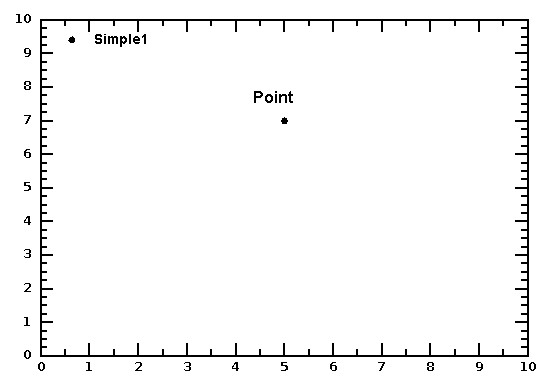## Showing shapes and objects

You can show data and functions together with different 2D objects. Here is a simple example that shows a histogram, a data set in X-Y and 3 ellipses:

Here is the output of this example: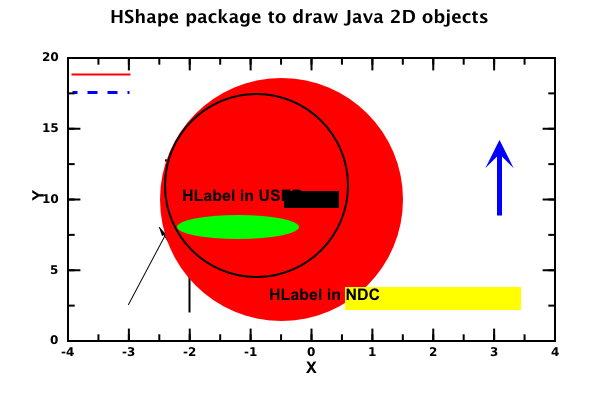# Post editingjhplot.HPlotJa can be used to edit figures. For example, one can make inserts if one creates 2 plotting pads and then one can edit the pads using the "mouse-click" fashion. For example, run this script:

Then edit the figure (increase the size of one pad, and then drag the other one):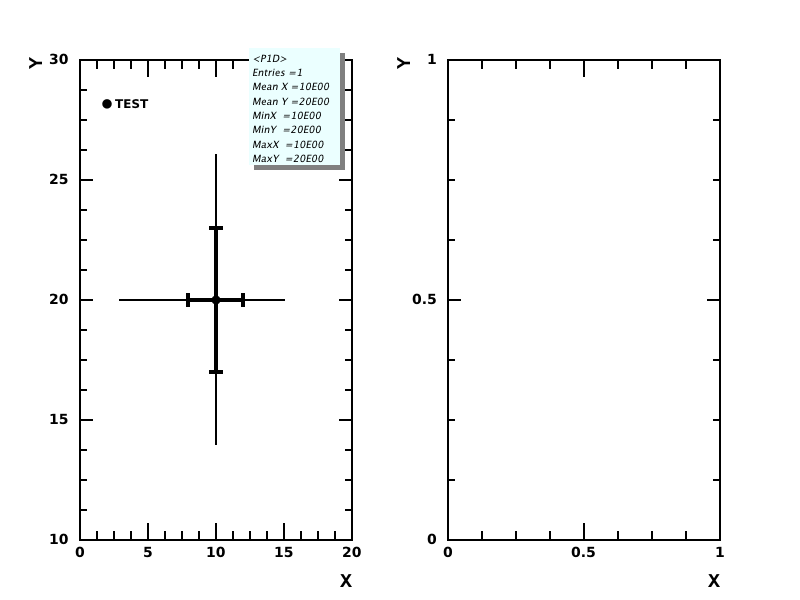Similarly, one can achieve the same using the method "setPad()" where you can specify the location and the sizes of the plot regions The script below creates 2 plotting pads. The second pad is located inside the first one. Then you can plot data as usual, navigating to certain pads using the "cd(i,j)" method.

from jhplot  import  *
c1 = HPlotJa("Canvas",600,400,2,1)
c1.visible(1)

# change pad positions and sizes
c1.setPad(2,1,0.5, 0.14, 0.3,0.3)

# Interactive plottingjhplot.HPlotJas canvas represents a way to prepare all objects for plotting, fitting and rebinning of data.

# Polar coordinates

For the polar coordinates, use thejhplot.HChart canvas. A small code below shows ho to show a dataset filled from the X-Y arrayjhplot.P1D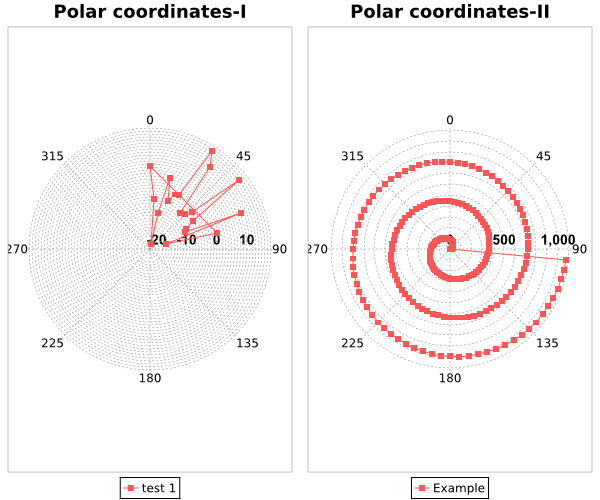# Example with 2D plot regions

In the example below, we create 4 plot regions (2 by 2) and plot functions and a histogram (see DMelt:DataAnalysis/3_Histograms). Then we export the plot to EPS file for inclusion to a LaTeX document

The output of this script is shown below: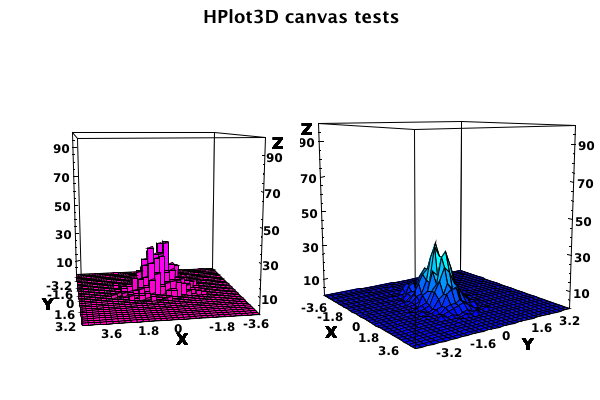Use setAutoRange() if you want X-Y-Z ranges taken from the function definitions. Otherwise, use "setRange()" to fix ranges. Here is another example showing 2D plots with data:

The output is shown below: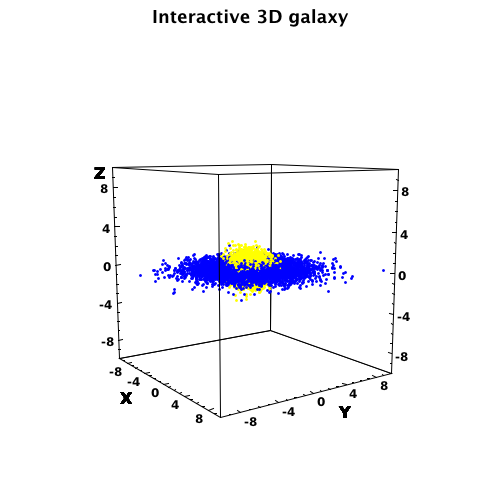# Embedding in JFrame

It is possible to embed DataMelt canvases in Javajava.swing.JFrame, so you can build an application with custom buttons. Here is an example:

# Using GROOT

DMelt includes GROOT package developed at JLab. Its syntax reminds the PyROOT environment. You can find example in Jython and Java here:

# Density plots

You can also make density plots in which color represent density (or values). Look at this rather comprehensive example which shows how to plot F2D functions or 2D histograms (H2D) using several pads: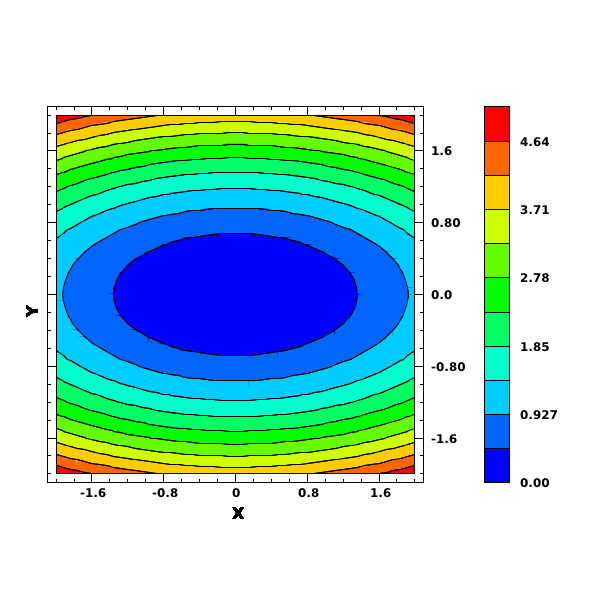# Waterloo scientific charts

DataMelt allows to access reach Waterloo scientific graphics package implemented in Java. The original web page is . However, DataMelt only used the base of the Waterloo package. A support for Groovy and Jython is done via the internal DataMelt api.

The API of these Java libraries for scientific plotting can be foundWaterloo graphics package. Let us give a small example of X-Y plot:

This plot shows the function x*x as shown below: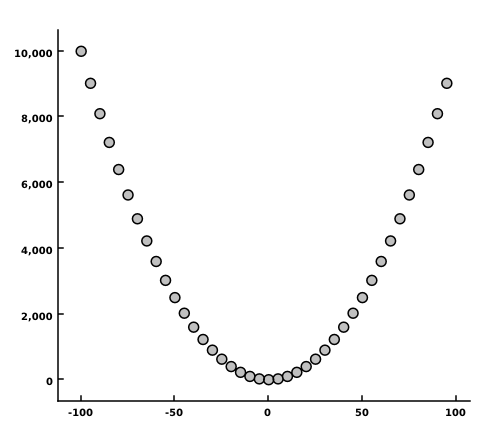# XChart scientific charts

DataMelt supports XChart library  and optimizes it for Jython and Groovy. You can find the API [API]

Let us give a small Jython example of a X-Y plot:

This plot creates high-quality image like this: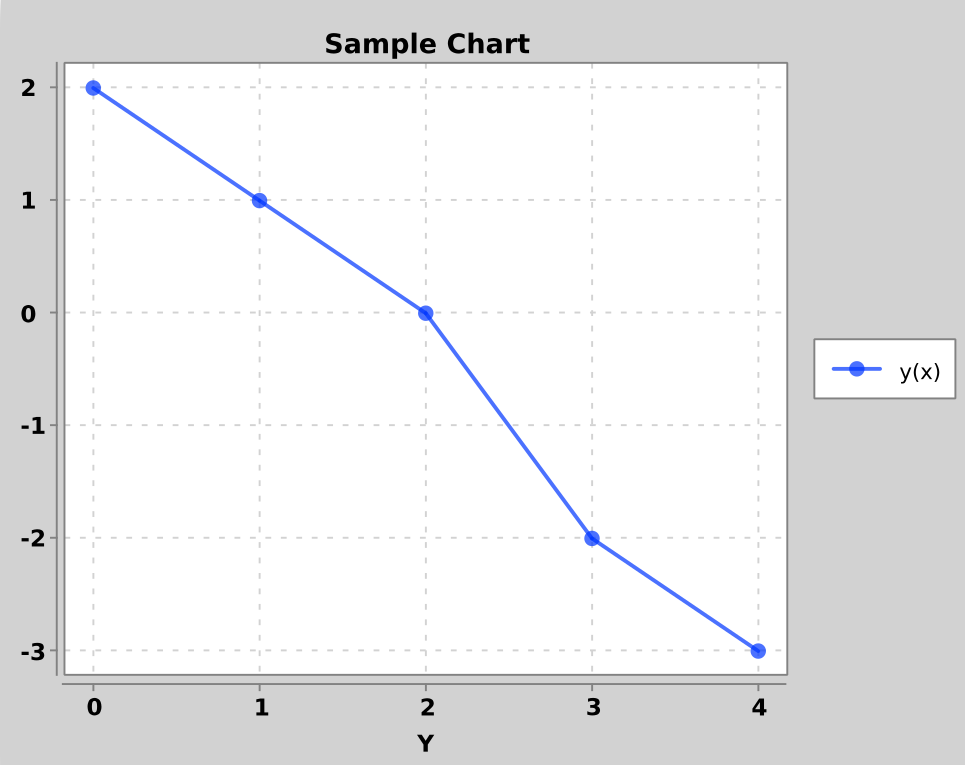The XChart library can create the following plots:

• Line charts
• Scatter charts
• Area charts
• Bar charts
• Histogram charts
• Pie charts
• Donut charts
• Bubble charts
• Stick charts
• Dial charts
• OHLC charts
• Box charts
• Heat maps
• Error bars
• Logarithmic axes
• Number, Date and Category X-Axis
• Multiple series
• User-defined axes range
• Definable legend placement

All images can be created in PDF, PNG, JPG, BMP and GIF. The possibility of CSV import and export is also included.

# Other charts

DataMelt can be used to plot many other types of charts, such as Candlestick chart. You can also create fully customizable layouts for 2D charts.